Maths-
General
Easy

Question

# The number of different seven digit numbers that can be written using only the three digits 1, 2 and 3 with the condition that the digit 2 occurs twice in each number is-

## 7P2 . 25    7C2 . 25    7C2 . 52    None of theseHint:

## The correct answer is: 7C2 . 25

### Detailed SolutionWe are given the number of different seven-digit numbers that can be written using only three digits 1,2 and 3. Therefore,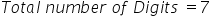We are given that the digit two occurs exactly twice in each number.Thus, the digit two occurs twice in the seven digit number.Now, we will find the number of ways of arrangement of the digit two in the seven digit number by using combination.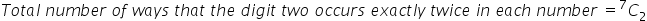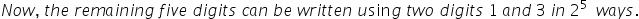We will now find the total number of seven digit number by multiplying the number of ways of arrangement in both the cases. Therefore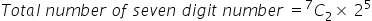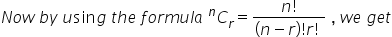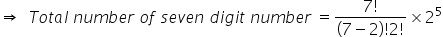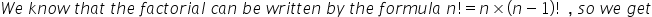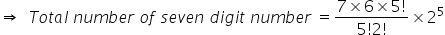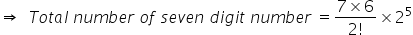Simplifying the expression, we get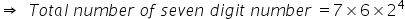Multiplying the terms, we get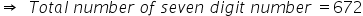Therefore, the number of different seven-digit numbers that can be written using only three digits 1,2 and 3 is 672.

We know that there is not much difference between permutation and combination. Permutation is the way or method of arranging numbers from a given set of numbers such that the order of arrangement matters. Whereas combination is the way of selecting items from a given set of items where order of selection doesn’t matter. Both the word combination and permutation is the way of arrangement. Here, we will not use permutation because the order of toys is not necessary.#### With Turito Foundation.#### Get an Expert Advice From Turito.